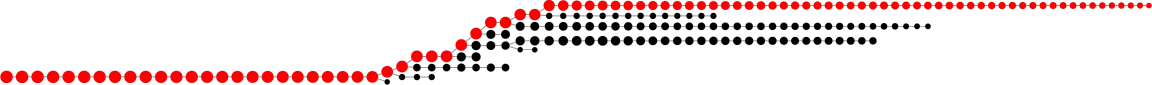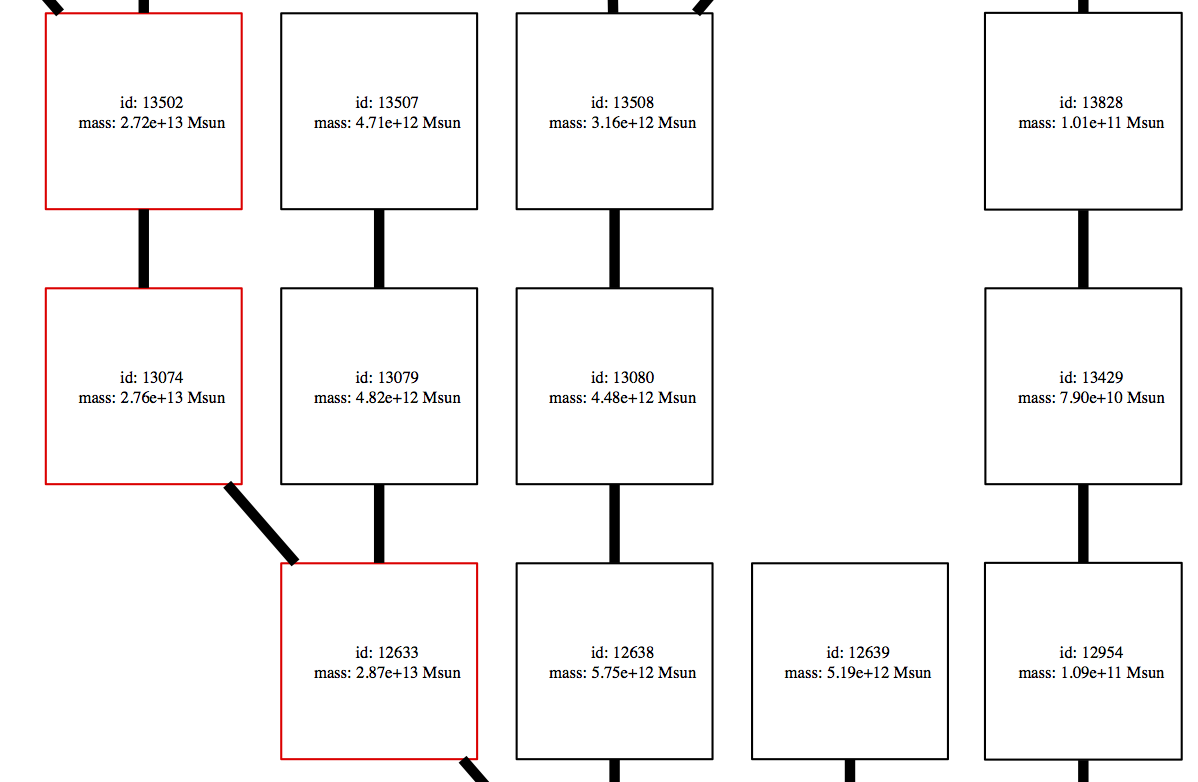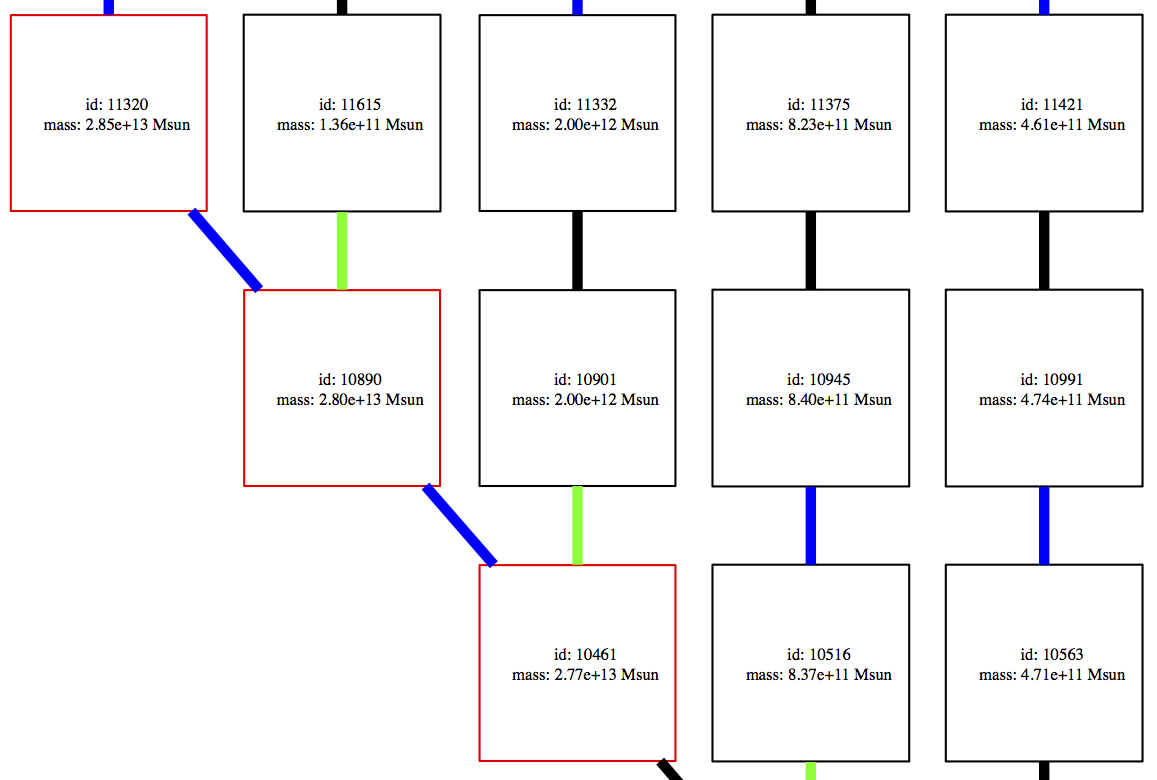# Plotting Merger Trees¶

Some relatively simple visualizations of merger trees can be made with the `TreePlot` command.

Making merger tree plots with `ytree` requires the pydot and graphviz packages. `pydot` can be installed with `pip` and the graphviz website provides a number of installation options.

## Making Tree Plots¶

The `TreePlot` command can be used to create a digraph depicting halos as filled circles with sizes proportional to their mass. The main progenitor line will be colored red.

```>>> import ytree
...                hubble_constant=0.7)
>>> p = ytree.TreePlot(a, dot_kwargs={'rankdir': 'LR', 'size': '"12,4"'})
>>> p.save('tree.png')
```### Plot Modifications¶

Four `TreePlot` attributes can be set to modify the default plotting behavior. These are:

```>>> import ytree
...                hubble_constant=0.7)
>>> p = ytree.TreePlot(a, dot_kwargs={'rankdir': 'LR', 'size': '"12,4"'})
>>> p.min_mass_ratio = 0.01
>>> p.save('tree_small.png')
```### Customizing Node Appearance¶

The appearance of the nodes can be customized by providing a function that returns a dictionary of keywords that will be used to create the `pydot` node. This should accept a single argument that is a `TreeNode` object representing the halo to be plotted. For example, the following function will add labels of the halo id and mass and make the node shape square. It will also color the most massive progenitor red.

```def my_node(halo):
prog = list(halo.find_root()['prog', 'uid'])
if halo['uid'] in prog:
color = 'red'
else:
color = 'black'

label = \
"""
id: %d
mass: %.2e Msun
""" % (halo['uid'], halo['mass'].to('Msun'))

my_kwargs = {"label": label, "fontsize": 8,
"shape": "square", "color": color}
return my_kwargs
```

This function is then provided with the node_function keyword.

```>>> p = ytree.TreePlot(tree, dot_kwargs={'rankdir': "BT"},
...                    node_function=my_node)
>>> p.save('tree_custom_node.png')
```### Customizing Edge Appearance¶

The edges of the plot are the lines connecting each of the nodes. Similar to the nodes, their appearance can be customized by providing a function that returns a dictionary of keywords that will be used to create the `pydot` edge. This should accept two `TreeNode` arguments representing the ancestor and descendent halos being connected by the edge. The example below colors the edges blue when the descendent is less massive than its ancestor and green when the descendent is more than 10 times more massive than its ancestor.

```def my_edge(ancestor, descendent):
if descendent['mass'] < ancestor['mass']:
color = 'blue'
elif descendent['mass'] / ancestor['mass'] > 10:
color = 'green'
else:
color = 'black'

my_kwargs = {"color": color, "penwidth": 5}
return my_kwargs
```

This function is then provided with the edge_function keyword.

```>>> p = ytree.TreePlot(tree, dot_kwargs={'rankdir': "BT"},
...                    node_function=my_node,
...                    edge_function=my_edge)
>>> p.save('tree_custom_edge.png')
```### Supported Output Formats¶

Plots can be saved to any format supported by `graphviz` by giving a filename with the appropriate extension. See here for a list of currently supported formats.